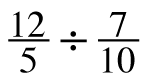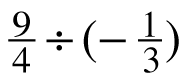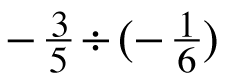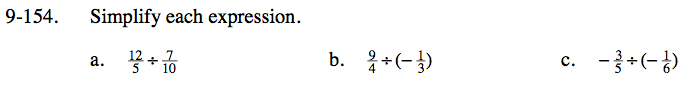### Home > CC3 > Chapter 9 > Lesson 9.2.7 > Problem9-154

9-154.
1. Simplify each expression. Homework Help ✎

1.2.3.Dividing by a fraction is the same as multiplying by its reciprocal.

$\frac{12}{5}\left(\frac{10}{7}\right)$

Simplifying at the beginning is easier than simplifying after multiplying.

$\frac{12}{1}\left(\frac{2}{7}\right)$

$\frac{24}{7}$

See the help for part (a).

$-\frac{27}{4}$

See the help for part (a).